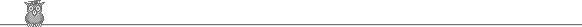### 27th USAMO 1998Problem A2

Two circles are concentric. A chord AC of the outer circle touches the inner circle at Q. P is the midpoint of AQ. A line through A intersects the inner circle at R and S. The perpendicular bisectors of PR and CS meet at T on the line AC. What is the ratio AT/TC?

Solution

We have AR·AS = AQ2 = AQ/2 2AQ = AP·AC, so ARP and ACS are similar, so ∠ACS = ∠ARP, so PRSC is cyclic. Hence T must be the center of its circumcircle and must also lie on the perpendicular bisector of CP. Hence it must be the midpoint of CP. So CT = 3/8 CA and hence AT/TC = 5/3.

However, that is not quite all. If CS is parallel to PR, then their perpendicular bisectors coincide and both pass through A. So one could also regard A as a possible position for T.